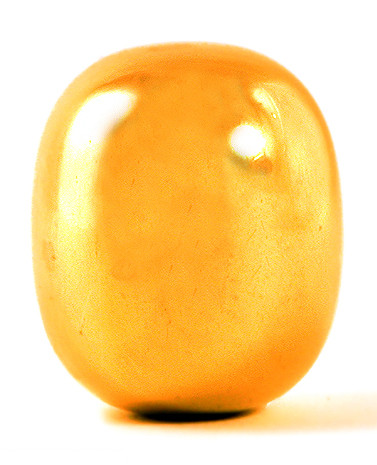# Stability of a superegg

Three weeks ago I wrote about supereggs, a shape popularized by Piet Hein.One aspect of supereggs that I did not address is their stability. Looking at the photo above, you could imagine that if you gave the object a slight nudge it would not fall over. Your intuition would be right: supereggs are stable.

More interesting than asking whether supereggs are stable is to aks how stable they are. An object is stable if for some ε > 0, a perturbation of size less than ε will not cause the object to fall over.

All supereggs are stable, but the degree of stability decreases as the ratio of height to width increases: the taller the superegg, the easier it is to knock over.

How can we quantify stability? An object is stable if its center of curvature, measured at the point of contact with a horizontal surface, is above its center of mass.

For a sphere, the center of curvature is exactly the center of mass. If we modify the sphere to make it slightly flatter at the point of contact, the center of curvature will move above the center of mass and the modified sphere will be stable. On the other hand, if we made the sphere slightly more curved at the point of contact, the center of curvature would move below the center of mass and the object would be unstable.

The center of curvature is the center of the sphere that best approximates a surface at a point. If our object is a sphere, the hypothetical sphere defining the curvature is the object itself. For an object flatter on bottom than a sphere, the sphere defining center of curvature is larger than the object. The flatter the object, the larger the approximating sphere.

The superegg has zero curvature at the bottom, and so its center of curvature is at infinity. But if you push the superegg slightly, the part touching the horizontal surface is no longer the exact center, the curvature is slightly positive, and so the radius of curvature is finite. The center of mass also moves up slightly as the superegg rocks to the side.

So the center of curvature moves down and the center of mass moves up. At what point do they cross and the object becomes unstable?

## Solution outline

The equation of the surface of the superegg iswhere p > 2 (a common choice is p = 5/2) and h > 1 (a common choice is h = 4/3).

If we tilt the superegg so that its axis of symmetry now makes a small angle θ with the z-axis, we have to answer several questions.

1. Where does the center of mass go?
2. What is the new point of contact with the horizontal surface?
3. Where is the center of curvature?
4. Is the center of curvature above or below the center of mass?

It’s easier to imagine lifting the superegg up from the surface, rotating it in the air, then lowering it to the surface. That way you don’t have to describe how the superegg rocks.

The superegg is radially symmetric about the vertical axis, so without loss of generality you can imagine the problem is limited to the xz plane.

It’s messy to work out the details, but in principle you could work out how much the superegg can be perturbed and return to its original position. You know a priori that the result will be a decreasing function of h and p.